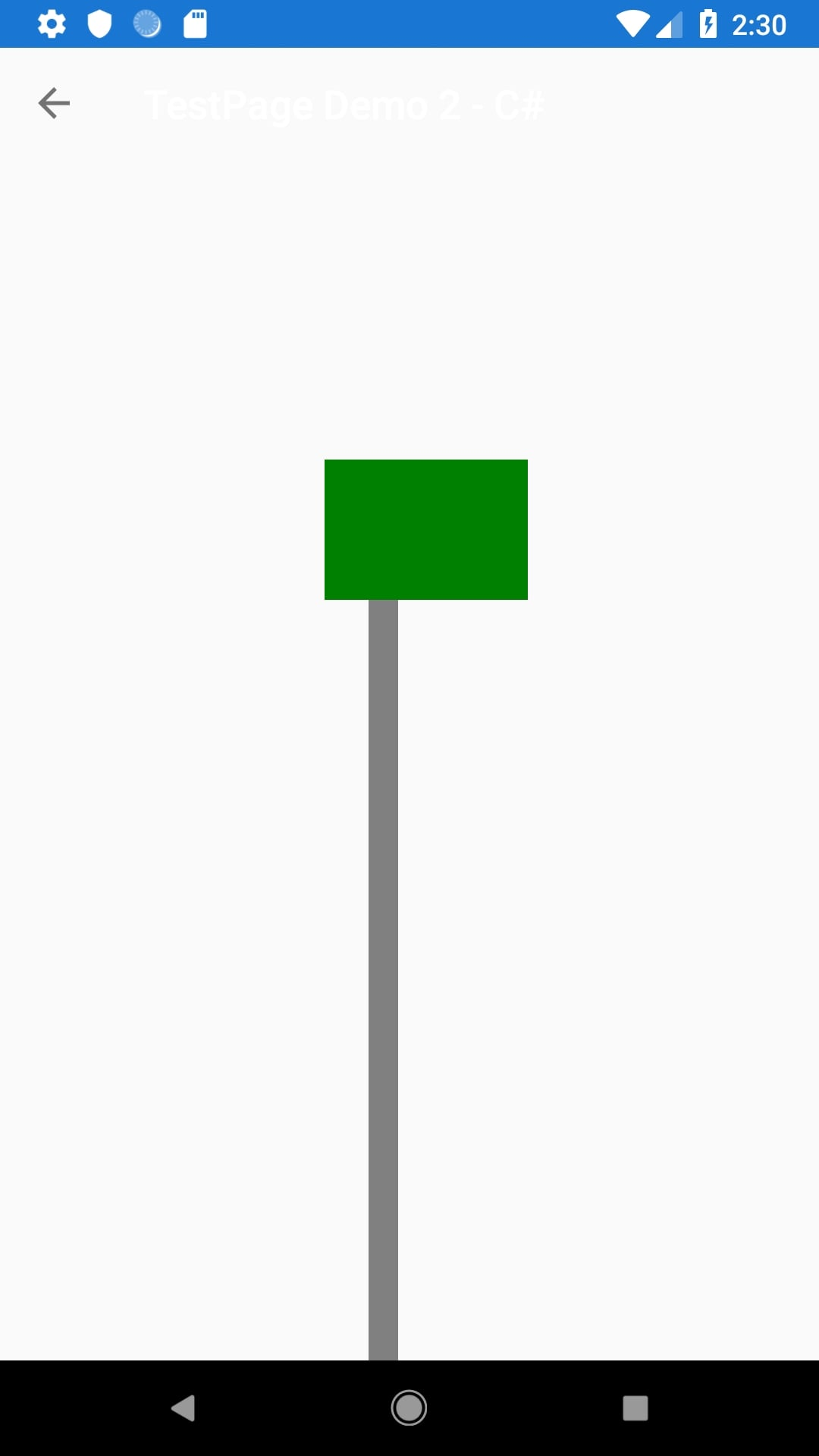# What's the c# quivalent of this Xaml?

<Image x:Name="TargetImage"
RelativeLayout.YConstraint="{ConstraintExpression Type=RelativeToView, ElementName=ZXingScannerView1, Property=Y, Constant={StaticResource TargetYConstant}}"
RelativeLayout.XConstraint="{ConstraintExpression Type=RelativeToView, ElementName=ZXingScannerView1, Property=X, Constant={StaticResource TargetXConstant}, Factor=0.80}">

Where TargetYConstant and TargetXConstant are variables in ResourceDictionary Keys in App.Xaml

• Member, Xamarin Team Xamurai

@toyins
I have created a simple demo, which achieve the same function in C# and Xaml.
Xaml code

``` <ContentPage xmlns="http://xamarin.com/schemas/2014/forms"
xmlns:x="http://schemas.microsoft.com/winfx/2009/xaml"
Title="Test Page Demo - XAML"
x:Class="LayoutSamples.TestPage">
<ContentPage.Content>
<RelativeLayout HorizontalOptions="FillAndExpand" VerticalOptions="FillAndExpand">
<BoxView Color="Gray" WidthRequest="15" x:Name="ZXingScannerView1"
RelativeLayout.HeightConstraint="{ConstraintExpression Type=RelativeToParent, Property=Height, Factor=.75}"
RelativeLayout.XConstraint= "{ConstraintExpression Type=RelativeToParent, Property=Width, Factor = .45}"
RelativeLayout.YConstraint="{ConstraintExpression Type=RelativeToParent, Property=Height, Factor = .25}" />
<Image BackgroundColor="Green"
RelativeLayout.HeightConstraint="{ConstraintExpression Type=RelativeToParent, Property=Height, Factor=.10,
Constant=10}"
RelativeLayout.WidthConstraint="{ConstraintExpression Type=RelativeToParent, Property=Width, Factor=.2,
Constant=20}"
RelativeLayout.XConstraint= "{ConstraintExpression Type=RelativeToView, ElementName=ZXingScannerView1,
Property=X, Constant=15,Factor= .8}"
RelativeLayout.YConstraint="{ConstraintExpression Type=RelativeToView, ElementName=ZXingScannerView1,
Property=Y, Constant=0}" />
</RelativeLayout>
</ContentPage.Content>
```

C# code

public class TestPage : ContentPage
{
public float x { get; set; }
public float y { get; set; }

```    Image image;
RelativeLayout layout;
BoxView grayBox;// you can define to `ZXingScannerView1`

public TestPage2 ()
{
Title = "TestPage Demo 2 - C#";
layout = new RelativeLayout();

grayBox = new BoxView { Color = Color.Gray, WidthRequest = 15 };
image = new Image { WidthRequest = 200,HeightRequest =200,BackgroundColor=Color.Green};

return parent.Width * .45;
}), Constraint.RelativeToParent((parent) => {
return parent.Height * .25;
}), null, Constraint.RelativeToParent((parent) => {
return parent.Height * .75;
}));

layout.Children.Add(image, Constraint.RelativeToView(grayBox, (Parent, sibling) => {
return (sibling.X * .8) + 15;   //  Factor=0.80
}), Constraint.RelativeToView(grayBox, (parent, sibling) => {
return sibling.Y;
}), Constraint.RelativeToParent((parent) => {
return (parent.Width * .2) + 20;
}), Constraint.RelativeToParent((parent) => {
return (parent.Height * .1) + 10;
}));
Content = layout;
}
}
```

The effect image isNote:
1. `ElementName` – the name of the view that the constraint is relative to.
2. I didn't use TargetYConstant and TargetXConstant, you can replace with them.

• Member, Xamarin Team Xamurai
edited March 2019

@toyins
Have you got the solution?